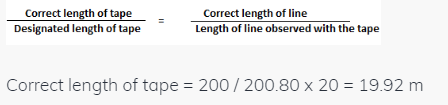Courses

# The true length of a line is known to be 200 m. when this is measured with a 20 m tape, the length is 200.80 m. The correct length of the 20 m tape isa)19.92 mb)19.98 mc)20.04 md)20.08 mCorrect answer is option 'A'. Can you explain this answer? Related Test: Test: Civil Engineering (SSC JE) Technical - 2

## Civil Engineering (CE) QuestionRavi Kalra Aug 02, 2018
True length = 200 m. tape length= 20 m measured length= 200.80 m Error = 200.80 - 200 = .80 m because tape will be used 10 times for measuring actual length thatswhy .80 will be divided in 10 parts which will be .08 so tape length would be 20 - .08 =19.92 m.Aakash Pandey Sep 19, 2018Real World Feb 27, 2019
19.92m